# Selina Solutions Concise Mathematics Class 6 Chapter 11:Ratio Exercise 11(B)

Selina Solutions Concise Mathematics Class 6 Chapter 11 Ratio Exercise 11(B) provides students the easy way of solving tricky problems in a short duration. By referring to solutions PDF, students can solve exercise wise problems with ease, at any time. Subject matter experts at BYJU’ S have designed the solutions in an interesting way, in order to boost self confidence among students in Mathematics. For better understanding of the concepts discussed in this exercise, students can follow Selina Solutions Concise Mathematics Class 6 Chapter 11 Ratio Exercise 11(B) PDF links, which are given here.

## Selina Solutions Concise Mathematics Class 6 Chapter 11: Ratio Exercise 11(B) Download PDF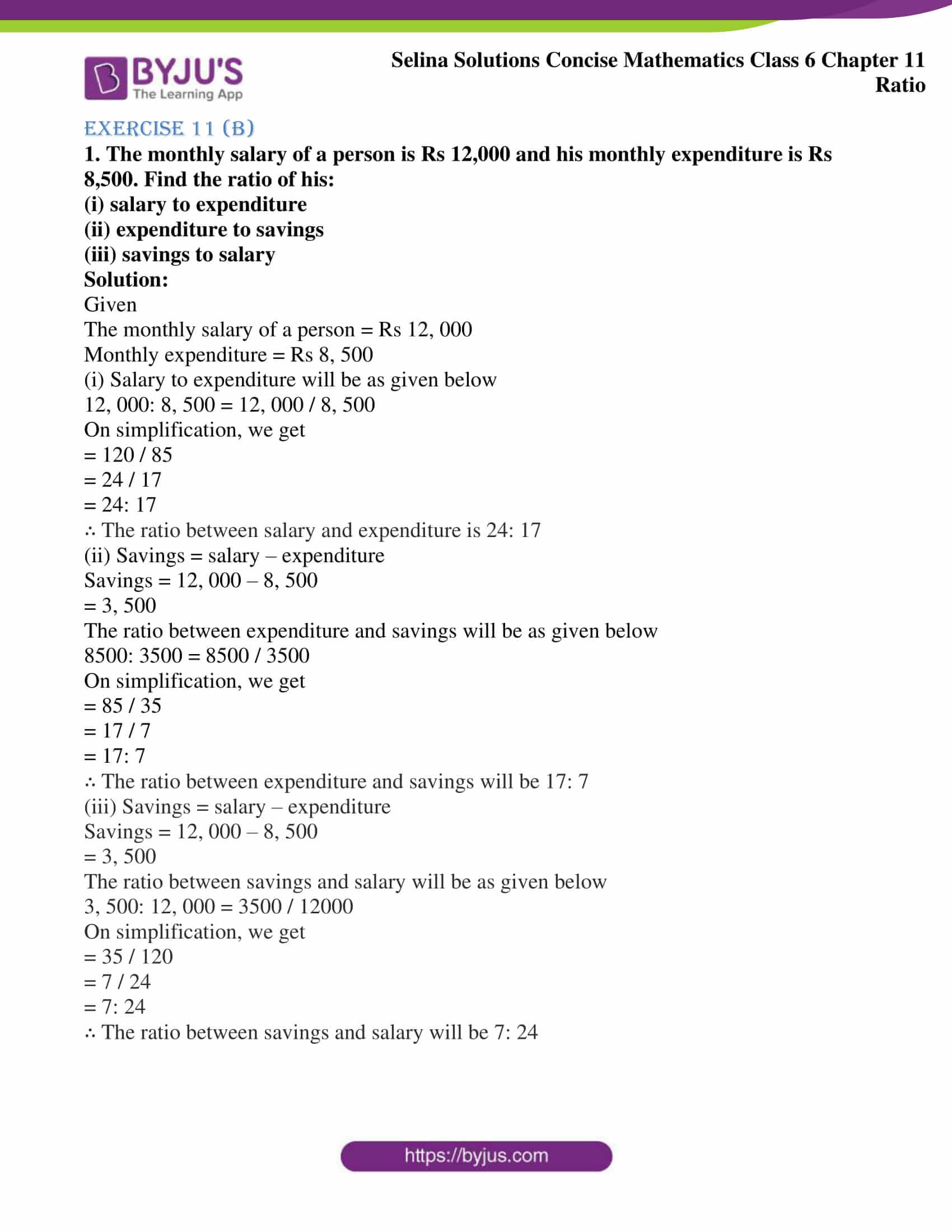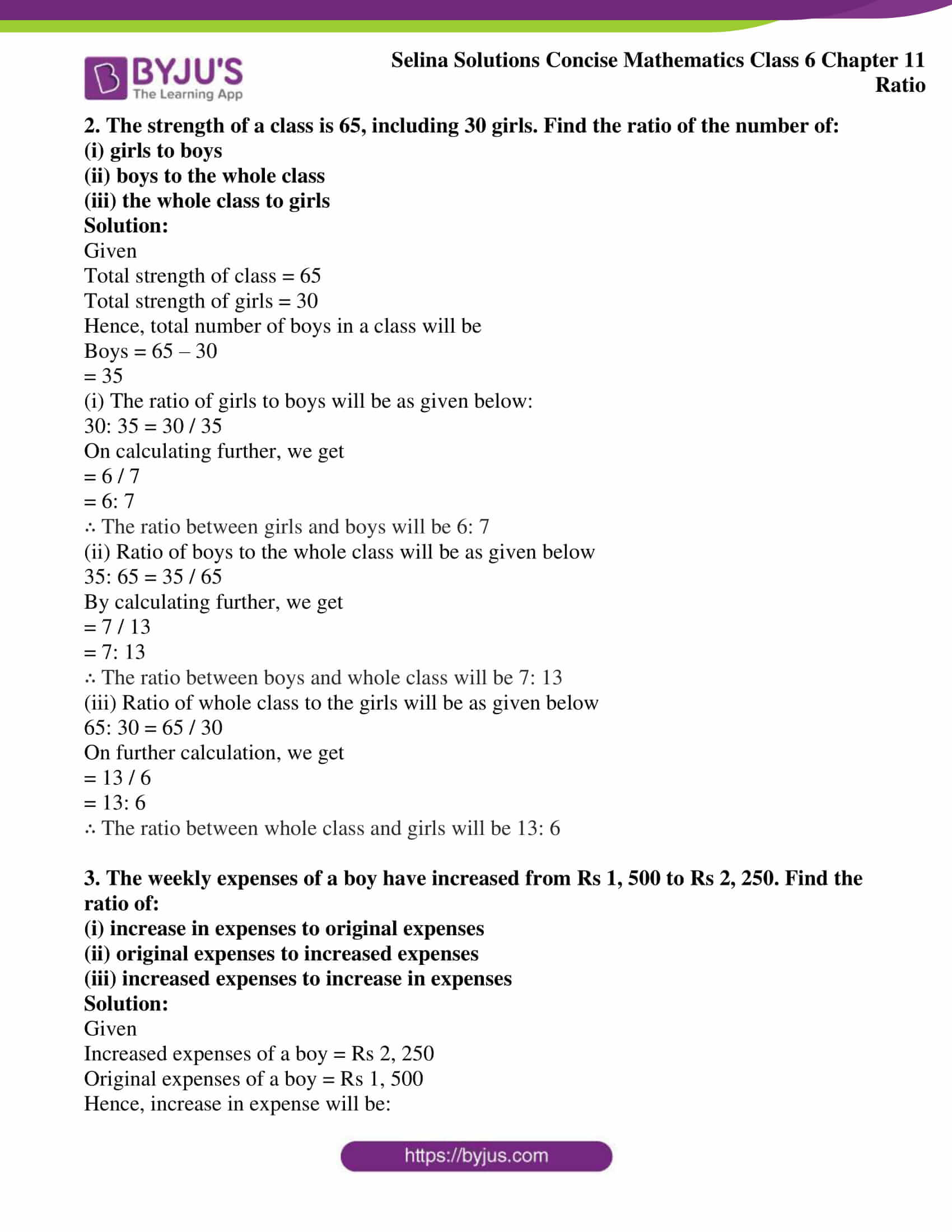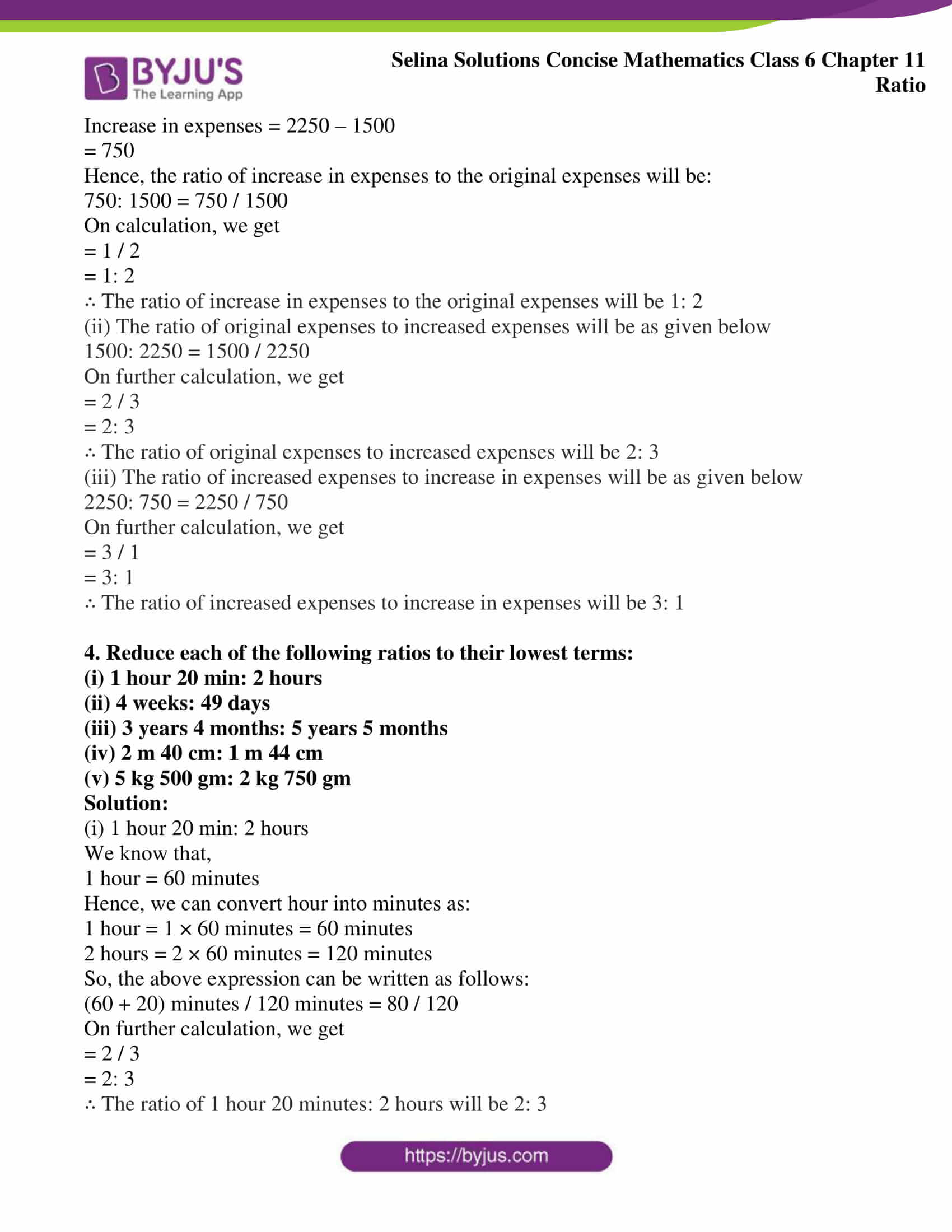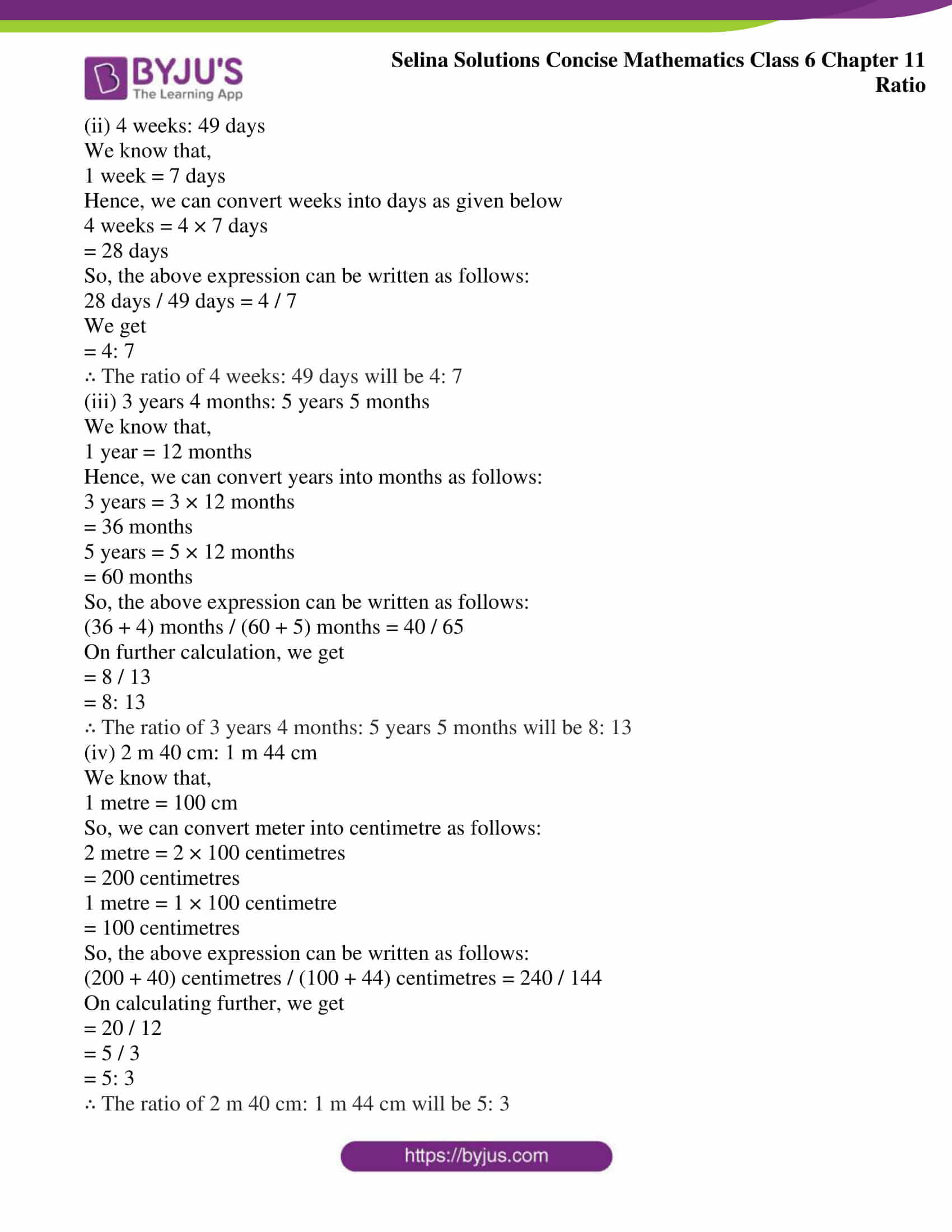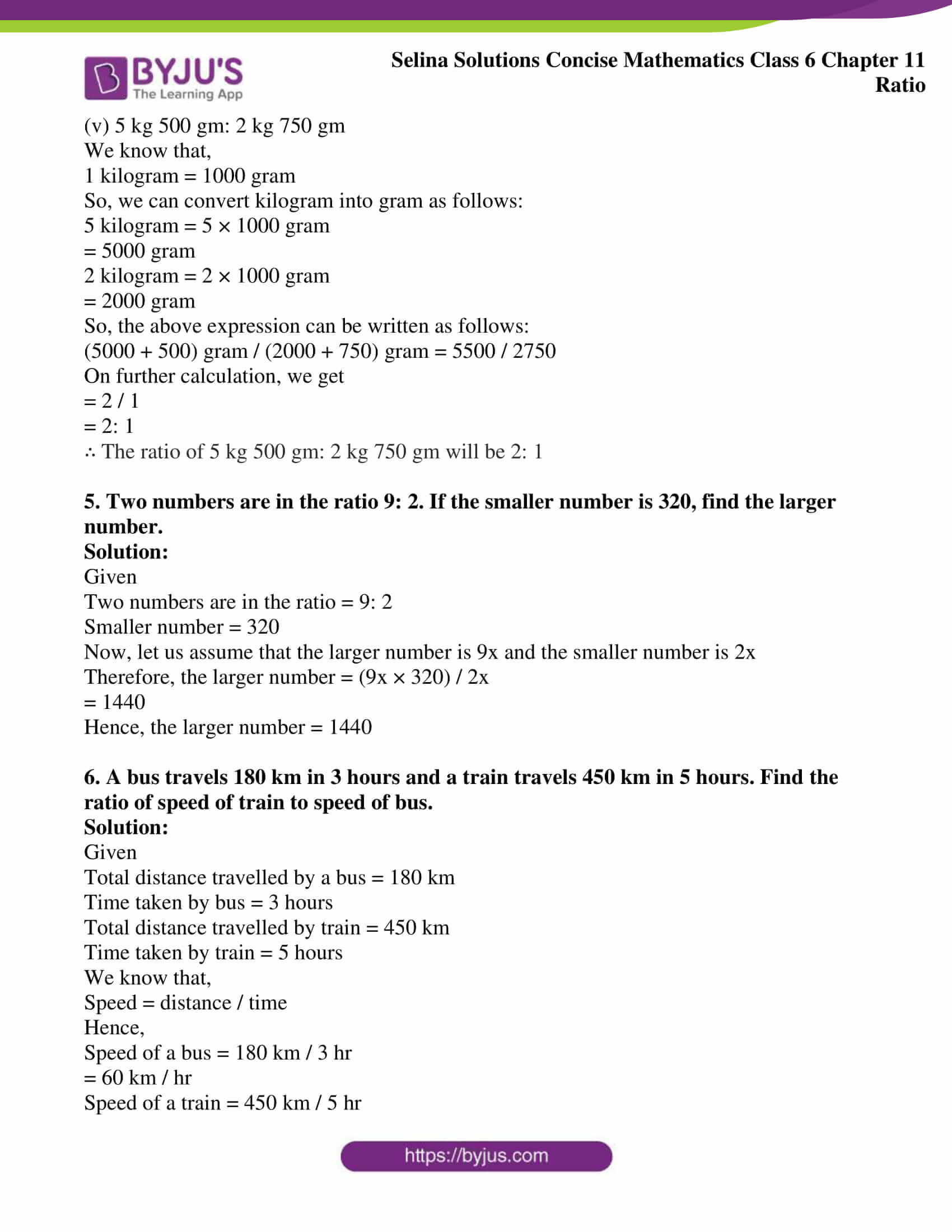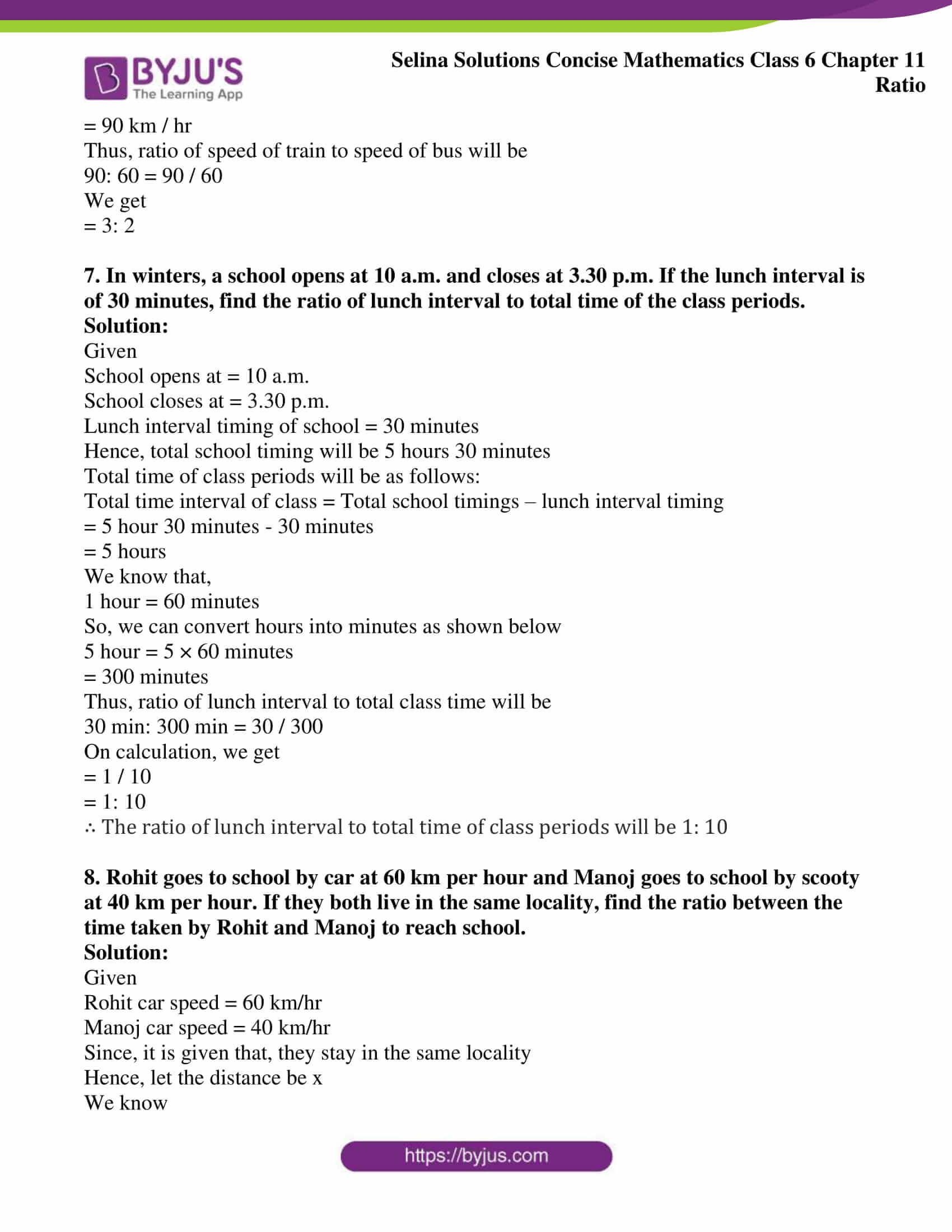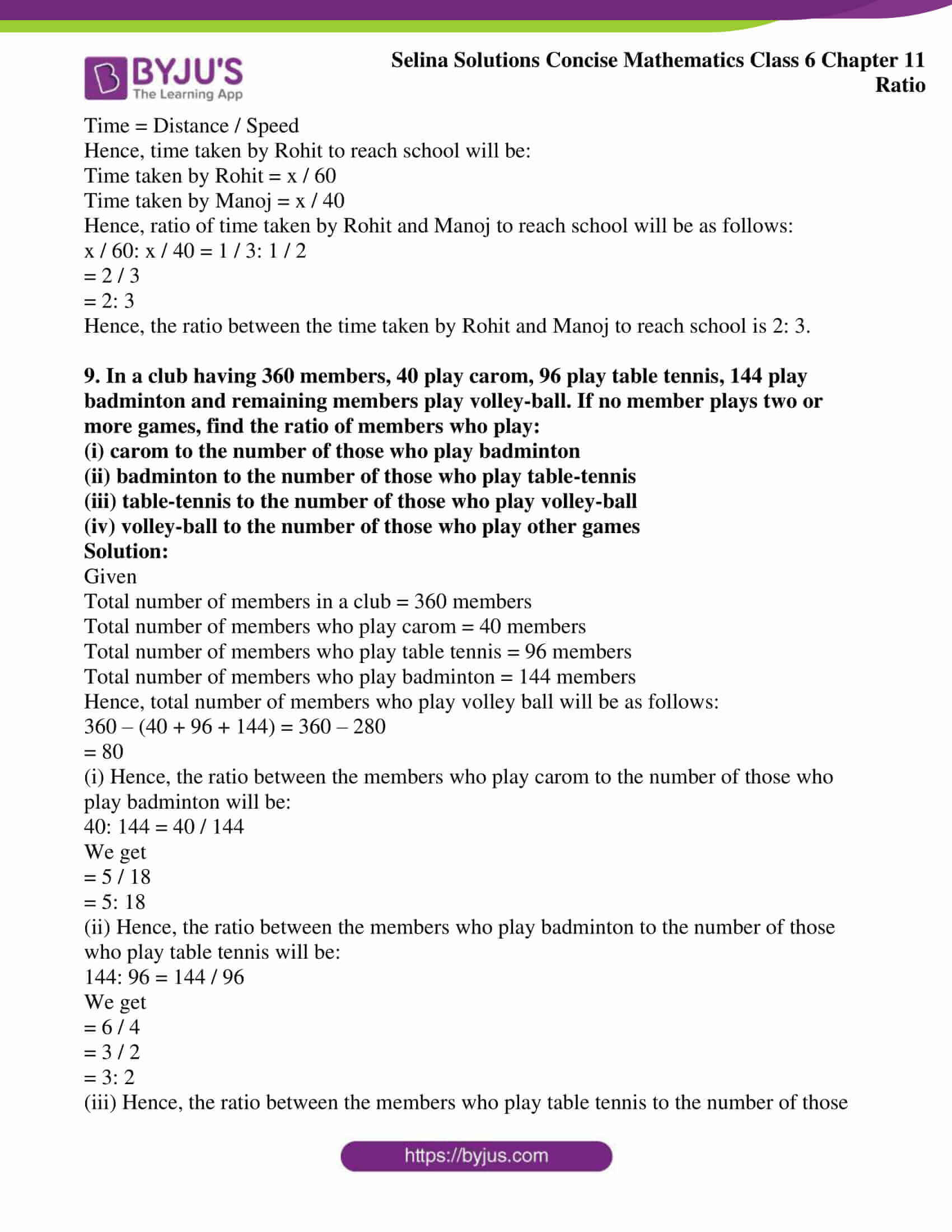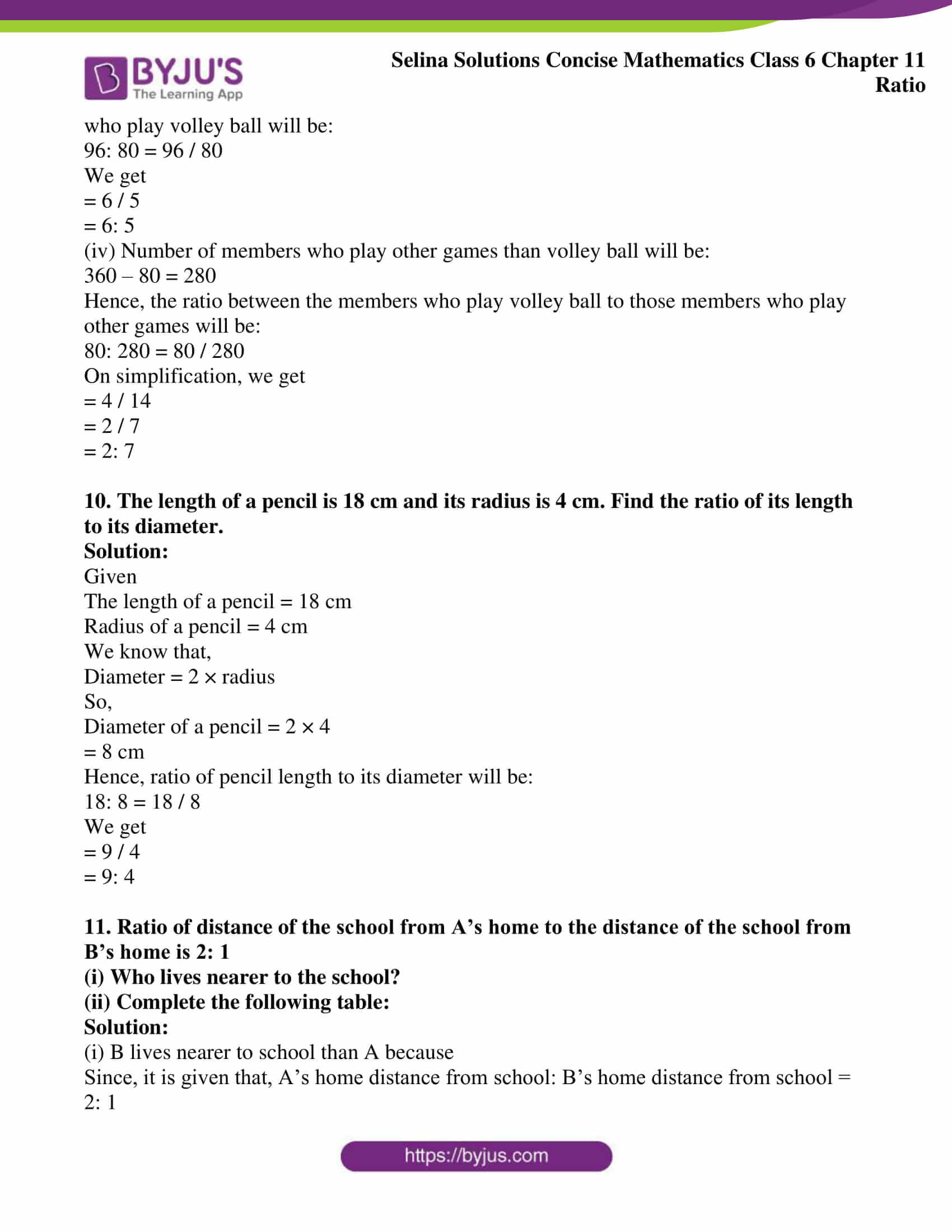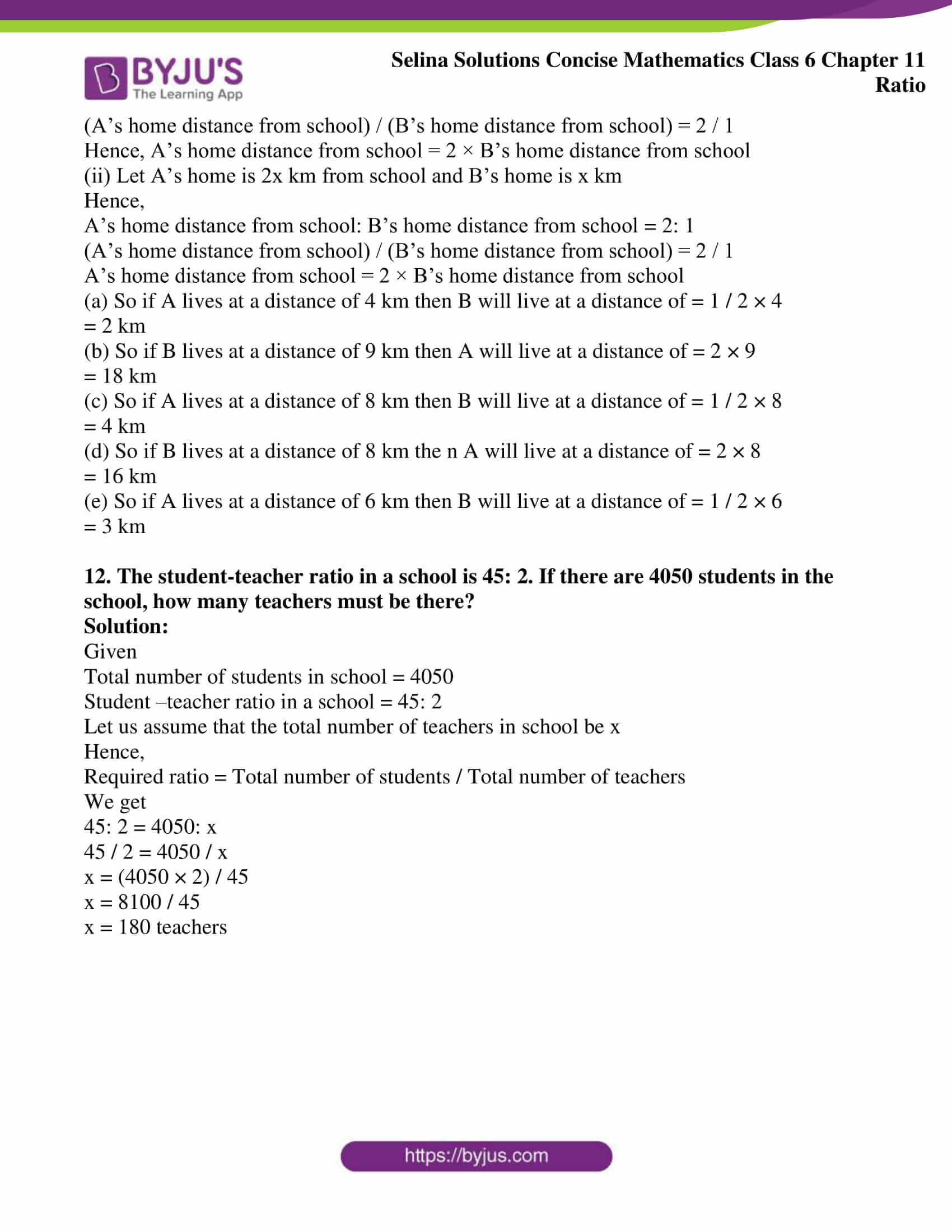### Access other exercises of Selina Solutions Concise Mathematics Class 6 Chapter 11: Ratio

Exercise 11(A) Solutions

Exercise 11(C) Solutions

Exercise 11(D) Solutions

### Access Selina Solutions Concise Mathematics Class 6 Chapter 11: Ratio Exercise 11(B)

#### Exercise 11 (B)

1. The monthly salary of a person is Rs 12,000 and his monthly expenditure is Rs 8,500. Find the ratio of his:

(i) salary to expenditure

(ii) expenditure to savings

(iii) savings to salary

Solution:

Given

The monthly salary of a person = Rs 12, 000

Monthly expenditure = Rs 8, 500

(i) Salary to expenditure will be as given below

12, 000: 8, 500 = 12, 000 / 8, 500

On simplification, we get

= 120 / 85

= 24 / 17

= 24: 17

∴ The ratio between salary and expenditure is 24: 17

(ii) Savings = salary – expenditure

Savings = 12, 000 – 8, 500

= 3, 500

The ratio between expenditure and savings will be as given below

8500: 3500 = 8500 / 3500

On simplification, we get

= 85 / 35

= 17 / 7

= 17: 7

∴ The ratio between expenditure and savings will be 17: 7

(iii) Savings = salary – expenditure

Savings = 12, 000 – 8, 500

= 3, 500

The ratio between savings and salary will be as given below

3, 500: 12, 000 = 3500 / 12000

On simplification, we get

= 35 / 120

= 7 / 24

= 7: 24

∴ The ratio between savings and salary will be 7: 24

2. The strength of a class is 65, including 30 girls. Find the ratio of the number of:

(i) girls to boys

(ii) boys to the whole class

(iii) the whole class to girls

Solution:

Given

Total strength of class = 65

Total strength of girls = 30

Hence, total number of boys in a class will be

Boys = 65 – 30

= 35

(i) The ratio of girls to boys will be as given below:

30: 35 = 30 / 35

On calculating further, we get

= 6 / 7

= 6: 7

∴ The ratio between girls and boys will be 6: 7

(ii) Ratio of boys to the whole class will be as given below

35: 65 = 35 / 65

By calculating further, we get

= 7 / 13

= 7: 13

∴ The ratio between boys and whole class will be 7: 13

(iii) Ratio of whole class to the girls will be as given below

65: 30 = 65 / 30

On further calculation, we get

= 13 / 6

= 13: 6

∴ The ratio between whole class and girls will be 13: 6

3. The weekly expenses of a boy have increased from Rs 1, 500 to Rs 2, 250. Find the ratio of:

(i) increase in expenses to original expenses

(ii) original expenses to increased expenses

(iii) increased expenses to increase in expenses

Solution:

Given

Increased expenses of a boy = Rs 2, 250

Original expenses of a boy = Rs 1, 500

Hence, increase in expense will be:

Increase in expenses = 2250 – 1500

= 750

Hence, the ratio of increase in expenses to the original expenses will be:

750: 1500 = 750 / 1500

On calculation, we get

= 1 / 2

= 1: 2

∴ The ratio of increase in expenses to the original expenses will be 1: 2

(ii) The ratio of original expenses to increased expenses will be as given below

1500: 2250 = 1500 / 2250

On further calculation, we get

= 2 / 3

= 2: 3

∴ The ratio of original expenses to increased expenses will be 2: 3

(iii) The ratio of increased expenses to increase in expenses will be as given below

2250: 750 = 2250 / 750

On further calculation, we get

= 3 / 1

= 3: 1

∴ The ratio of increased expenses to increase in expenses will be 3: 1

4. Reduce each of the following ratios to their lowest terms:

(i) 1 hour 20 min: 2 hours

(ii) 4 weeks: 49 days

(iii) 3 years 4 months: 5 years 5 months

(iv) 2 m 40 cm: 1 m 44 cm

(v) 5 kg 500 gm: 2 kg 750 gm

Solution:

(i) 1 hour 20 min: 2 hours

We know that,

1 hour = 60 minutes

Hence, we can convert hour into minutes as:

1 hour = 1 × 60 minutes = 60 minutes

2 hours = 2 × 60 minutes = 120 minutes

So, the above expression can be written as follows:

(60 + 20) minutes / 120 minutes = 80 / 120

On further calculation, we get

= 2 / 3

= 2: 3

∴ The ratio of 1 hour 20 minutes: 2 hours will be 2: 3

(ii) 4 weeks: 49 days

We know that,

1 week = 7 days

Hence, we can convert weeks into days as given below

4 weeks = 4 × 7 days

= 28 days

So, the above expression can be written as follows:

28 days / 49 days = 4 / 7

We get

= 4: 7

∴ The ratio of 4 weeks: 49 days will be 4: 7

(iii) 3 years 4 months: 5 years 5 months

We know that,

1 year = 12 months

Hence, we can convert years into months as follows:

3 years = 3 × 12 months

= 36 months

5 years = 5 × 12 months

= 60 months

So, the above expression can be written as follows:

(36 + 4) months / (60 + 5) months = 40 / 65

On further calculation, we get

= 8 / 13

= 8: 13

∴ The ratio of 3 years 4 months: 5 years 5 months will be 8: 13

(iv) 2 m 40 cm: 1 m 44 cm

We know that,

1 metre = 100 cm

So, we can convert meter into centimetre as follows:

2 metre = 2 × 100 centimetres

= 200 centimetres

1 metre = 1 × 100 centimetre

= 100 centimetres

So, the above expression can be written as follows:

(200 + 40) centimetres / (100 + 44) centimetres = 240 / 144

On calculating further, we get

= 20 / 12

= 5 / 3

= 5: 3

∴ The ratio of 2 m 40 cm: 1 m 44 cm will be 5: 3

(v) 5 kg 500 gm: 2 kg 750 gm

We know that,

1 kilogram = 1000 gram

So, we can convert kilogram into gram as follows:

5 kilogram = 5 × 1000 gram

= 5000 gram

2 kilogram = 2 × 1000 gram

= 2000 gram

So, the above expression can be written as follows:

(5000 + 500) gram / (2000 + 750) gram = 5500 / 2750

On further calculation, we get

= 2 / 1

= 2: 1

∴ The ratio of 5 kg 500 gm: 2 kg 750 gm will be 2: 1

5. Two numbers are in the ratio 9: 2. If the smaller number is 320, find the larger number.

Solution:

Given

Two numbers are in the ratio = 9: 2

Smaller number = 320

Now, let us assume that the larger number is 9x and the smaller number is 2x

Therefore, the larger number = (9x × 320) / 2x

= 1440

Hence, the larger number = 1440

6. A bus travels 180 km in 3 hours and a train travels 450 km in 5 hours. Find the ratio of speed of train to speed of bus.

Solution:

Given

Total distance travelled by a bus = 180 km

Time taken by bus = 3 hours

Total distance travelled by train = 450 km

Time taken by train = 5 hours

We know that,

Speed = distance / time

Hence,

Speed of a bus = 180 km / 3 hr

= 60 km / hr

Speed of a train = 450 km / 5 hr

= 90 km / hr

Thus, ratio of speed of train to speed of bus will be

90: 60 = 90 / 60

We get

= 3: 2

7. In winters, a school opens at 10 a.m. and closes at 3.30 p.m. If the lunch interval is of 30 minutes, find the ratio of lunch interval to total time of the class periods.

Solution:

Given

School opens at = 10 a.m.

School closes at = 3.30 p.m.

Lunch interval timing of school = 30 minutes

Hence, total school timing will be 5 hours 30 minutes

Total time of class periods will be as follows:

Total time interval of class = Total school timings – lunch interval timing

= 5 hour 30 minutes – 30 minutes

= 5 hours

We know that,

1 hour = 60 minutes

So, we can convert hours into minutes as shown below

5 hour = 5 × 60 minutes

= 300 minutes

Thus, ratio of lunch interval to total class time will be

30 min: 300 min = 30 / 300

On calculation, we get

= 1 / 10

= 1: 10

∴ The ratio of lunch interval to total time of class periods will be 1: 10

8. Rohit goes to school by car at 60 km per hour and Manoj goes to school by scooty at 40 km per hour. If they both live in the same locality, find the ratio between the time taken by Rohit and Manoj to reach school.

Solution:

Given

Rohit car speed = 60 km/hr

Manoj car speed = 40 km/hr

Since, it is given that, they stay in the same locality

Hence, let the distance be x

We know

Time = Distance / Speed

Hence, time taken by Rohit to reach school will be:

Time taken by Rohit = x / 60

Time taken by Manoj = x / 40

Hence, ratio of time taken by Rohit and Manoj to reach school will be as follows:

x / 60: x / 40 = 1 / 3: 1 / 2

= 2 / 3

= 2: 3

Hence, the ratio between the time taken by Rohit and Manoj to reach school is 2: 3.

9. In a club having 360 members, 40 play carom, 96 play table tennis, 144 play badminton and remaining members play volley-ball. If no member plays two or more games, find the ratio of members who play:

(i) carom to the number of those who play badminton

(ii) badminton to the number of those who play table-tennis

(iii) table-tennis to the number of those who play volley-ball

(iv) volley-ball to the number of those who play other games

Solution:

Given

Total number of members in a club = 360 members

Total number of members who play carom = 40 members

Total number of members who play table tennis = 96 members

Total number of members who play badminton = 144 members

Hence, total number of members who play volley ball will be as follows:

360 – (40 + 96 + 144) = 360 – 280

= 80

(i) Hence, the ratio between the members who play carom to the number of those who play badminton will be:

40: 144 = 40 / 144

We get

= 5 / 18

= 5: 18

(ii) Hence, the ratio between the members who play badminton to the number of those who play table tennis will be:

144: 96 = 144 / 96

We get

= 6 / 4

= 3 / 2

= 3: 2

(iii) Hence, the ratio between the members who play table tennis to the number of those who play volley ball will be:

96: 80 = 96 / 80

We get

= 6 / 5

= 6: 5

(iv) Number of members who play other games than volley ball will be:

360 – 80 = 280

Hence, the ratio between the members who play volley ball to those members who play other games will be:

80: 280 = 80 / 280

On simplification, we get

= 4 / 14

= 2 / 7

= 2: 7

10. The length of a pencil is 18 cm and its radius is 4 cm. Find the ratio of its length to its diameter.

Solution:

Given

The length of a pencil = 18 cm

Radius of a pencil = 4 cm

We know that,

So,

Diameter of a pencil = 2 × 4

= 8 cm

Hence, ratio of pencil length to its diameter will be:

18: 8 = 18 / 8

We get

= 9 / 4

= 9: 4

11. Ratio of distance of the school from A’s home to the distance of the school from B’s home is 2: 1

(i) Who lives nearer to the school?

(ii) Complete the following table:

Solution:

(i) B lives nearer to school than A because

Since, it is given that, A’s home distance from school: B’s home distance from school = 2: 1

(A’s home distance from school) / (B’s home distance from school) = 2 / 1

Hence, A’s home distance from school = 2 × B’s home distance from school

(ii) Let A’s home is 2x km from school and B’s home is x km

Hence,

A’s home distance from school: B’s home distance from school = 2: 1

(A’s home distance from school) / (B’s home distance from school) = 2 / 1

A’s home distance from school = 2 × B’s home distance from school

(a) So if A lives at a distance of 4 km then B will live at a distance of = 1 / 2 × 4

= 2 km

(b) So if B lives at a distance of 9 km then A will live at a distance of = 2 × 9

= 18 km

(c) So if A lives at a distance of 8 km then B will live at a distance of = 1 / 2 × 8

= 4 km

(d) So if B lives at a distance of 8 km the n A will live at a distance of = 2 × 8

= 16 km

(e) So if A lives at a distance of 6 km then B will live at a distance of = 1 / 2 × 6

= 3 km

12. The student-teacher ratio in a school is 45: 2. If there are 4050 students in the school, how many teachers must be there?

Solution:

Given

Total number of students in school = 4050

Student –teacher ratio in a school = 45: 2

Let us assume that the total number of teachers in school be x

Hence,

Required ratio = Total number of students / Total number of teachers

We get

45: 2 = 4050: x

45 / 2 = 4050 / x

x = (4050 × 2) / 45

x = 8100 / 45

x = 180 teachers